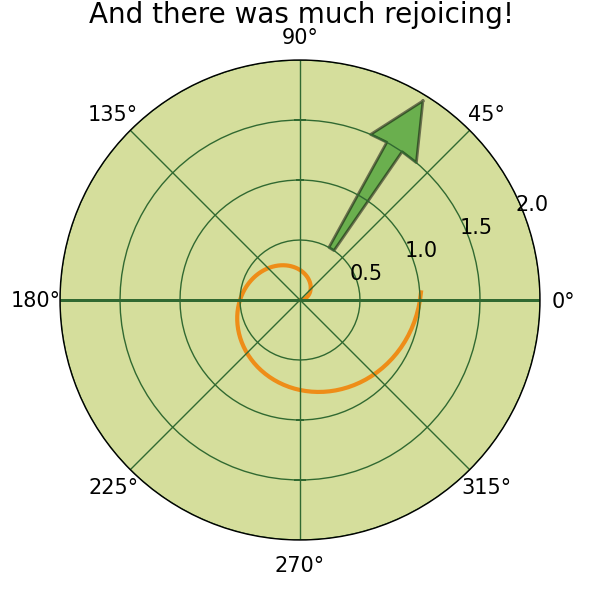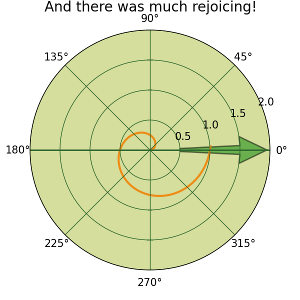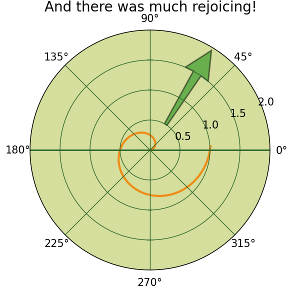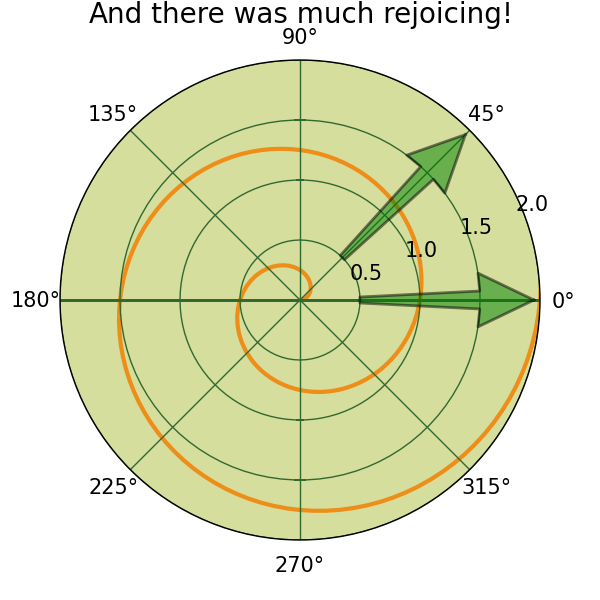# Arrows in polar plot at zero degree

Hello everybody,

I currently experience some problem with arrows in polar
plots.

Everything is fine, as long as the arrow does not cross
the zero line. Here is an example from the matplotlib
gallery
(http://matplotlib.sourceforge.net/examples/pylab_examples/polar_demo.html),
but with an arrow pointing at 45� outward.
To create the arrow I just added the following line:

arr = plt.arrow(45, 0.5, 0,1 , alpha = 0.5, width = 0.1,
edgecolor = 'black', facecolor = 'green',lw = 2)

You can find the complete source code at the end of the
mail.
If I want to point the arrow in zero direction,

arr = plt.arrow(0, 0.5, 0,1 , alpha = 0.5, width = 0.1,
edgecolor = 'black', facecolor = 'green',lw = 2)

there is only the silhouette of an arrow visible, but
nearly everthing seems to be green (as the arrow should
be). For me it seems as there are some problems with the
periodicity in polar plots.

Does anyone have an idea or a workaround?

Thank you very much in advance

Marie-Therese

···

---------------------------------------------------------------------------
Source code to reproduce the zero direction "arrow"

import matplotlib
import numpy as np
from matplotlib.pyplot import figure, show, rc, grid

# radar green, solid grid lines
rc('grid', color='#316931', linewidth=1, linestyle='-')
rc('xtick', labelsize=15)
rc('ytick', labelsize=15)

# force square figure and square axes looks better for
polar, IMO
width, height = matplotlib.rcParams['figure.figsize']
size = min(width, height)
# make a square figure
fig = figure(figsize=(size, size))
ax = fig.add_axes([0.1, 0.1, 0.8, 0.8], polar=True,
axisbg='#d5de9c')

r = np.arange(0, 3.0, 0.01)
theta = 2*np.pi*r
ax.plot(theta, r, color='#ee8d18', lw=3)
ax.set_rmax(2.0)
grid(True)

ax.set_title("And there was much rejoicing!", fontsize=20)
#This is the line I added:
arr = plt.arrow(0, 0.5, 0,1 , alpha = 0.5, width = 0.1,
edgecolor = 'black', facecolor = 'green',lw = 2)

show()

2009/10/15 Marie-Therese Horstmann <mhorstma@...2827...>:
I currently experience some problem with arrows in polar
plots.

---------------------------------------------------------------------------
Source code to reproduce the zero direction "arrow"

import matplotlib
import numpy as np
from matplotlib.pyplot import figure, show, rc, grid

# radar green, solid grid lines
rc('grid', color='#316931', linewidth=1, linestyle='-')
rc('xtick', labelsize=15)
rc('ytick', labelsize=15)

# force square figure and square axes looks better for
polar, IMO
width, height = matplotlib.rcParams['figure.figsize']
size = min(width, height)
# make a square figure
fig = figure(figsize=(size, size))
ax = fig.add_axes([0.1, 0.1, 0.8, 0.8], polar=True,
axisbg='#d5de9c')

r = np.arange(0, 3.0, 0.01)
theta = 2*np.pi*r
ax.plot(theta, r, color='#ee8d18', lw=3)
ax.set_rmax(2.0)
grid(True)

ax.set_title("And there was much rejoicing!", fontsize=20)
#This is the line I added:
arr = plt.arrow(0, 0.5, 0,1 , alpha = 0.5, width = 0.1,
edgecolor = 'black', facecolor = 'green',lw = 2)

arr = ax.arrow(0, 0.5, 0, 1, alpha = 0.5, width = 0.1 ...

show()

This works fine for me with matplotlib version 0.99.0 (see attached).
What version are you using?

import matplotlib
print matplotlib.__version__

Cheers,
Scott2009/10/15 Marie-Therese Horstmann <mhorstma@...2827...>:
I currently experience some problem with arrows in polar
plots.

---------------------------------------------------------------------------
Source code to reproduce the zero direction "arrow"

import matplotlib
import numpy as np
from matplotlib.pyplot import figure, show, rc, grid

# radar green, solid grid lines
rc('grid', color='#316931', linewidth=1, linestyle='-')
rc('xtick', labelsize=15)
rc('ytick', labelsize=15)

# force square figure and square axes looks better for
polar, IMO
width, height = matplotlib.rcParams['figure.figsize']
size = min(width, height)
# make a square figure
fig = figure(figsize=(size, size))
ax = fig.add_axes([0.1, 0.1, 0.8, 0.8], polar=True,
axisbg='#d5de9c')

r = np.arange(0, 3.0, 0.01)
theta = 2*np.pi*r
ax.plot(theta, r, color='#ee8d18', lw=3)
ax.set_rmax(2.0)
grid(True)

ax.set_title("And there was much rejoicing!", fontsize=20)
#This is the line I added:
arr = plt.arrow(0, 0.5, 0,1 , alpha = 0.5, width = 0.1,
edgecolor = 'black', facecolor = 'green',lw = 2)

arr = ax.arrow(0, 0.5, 0, 1, alpha = 0.5, width = 0.1 ...

show()

This works fine for me with matplotlib version 0.99.0 (see attached).
What version are you using?

import matplotlib
print matplotlib.__version__

Cheers,
ScottHi Marie-Therese,

I cannot reproduce your behaviour with current svn.
What version of matplotlib you are using?

I reattached your example (modified polar_demo.py) with small changes and
included the second arrow at 45. I cannot see any difference between the two
arrows (see attached arrow_in_polar_plot_at_zero_degree.png).

By the way I myself am a little bit confused by the label in degree and the

Kind regards,
Matthias···

On Thursday 15 October 2009 09:14:30 Marie-Therese Horstmann wrote:

Hello everybody,

I currently experience some problem with arrows in polar
plots.

Everything is fine, as long as the arrow does not cross
the zero line. Here is an example from the matplotlib
gallery
(http://matplotlib.sourceforge.net/examples/pylab_examples/polar_demo.html)
, but with an arrow pointing at 45° outward.
To create the arrow I just added the following line:

arr = plt.arrow(45, 0.5, 0,1 , alpha = 0.5, width = 0.1,
edgecolor = 'black', facecolor = 'green',lw = 2)

You can find the complete source code at the end of the
mail.
If I want to point the arrow in zero direction,

arr = plt.arrow(0, 0.5, 0,1 , alpha = 0.5, width = 0.1,
edgecolor = 'black', facecolor = 'green',lw = 2)

there is only the silhouette of an arrow visible, but
nearly everthing seems to be green (as the arrow should
be). For me it seems as there are some problems with the
periodicity in polar plots.

Does anyone have an idea or a workaround?

Thank you very much in advance

Marie-Therese

---------------------------------------------------------------------------
Source code to reproduce the zero direction "arrow"

import matplotlib
import numpy as np
from matplotlib.pyplot import figure, show, rc, grid

# radar green, solid grid lines
rc('grid', color='#316931', linewidth=1, linestyle='-')
rc('xtick', labelsize=15)
rc('ytick', labelsize=15)

# force square figure and square axes looks better for
polar, IMO
width, height = matplotlib.rcParams['figure.figsize']
size = min(width, height)
# make a square figure
fig = figure(figsize=(size, size))
ax = fig.add_axes([0.1, 0.1, 0.8, 0.8], polar=True,
axisbg='#d5de9c')

r = np.arange(0, 3.0, 0.01)
theta = 2*np.pi*r
ax.plot(theta, r, color='#ee8d18', lw=3)
ax.set_rmax(2.0)
grid(True)

ax.set_title("And there was much rejoicing!", fontsize=20)
#This is the line I added:
arr = plt.arrow(0, 0.5, 0,1 , alpha = 0.5, width = 0.1,
edgecolor = 'black', facecolor = 'green',lw = 2)

show()